# 2-torus

(diff) ← Older revision | Latest revision (diff) | Newer revision → (diff)
Jump to: navigation, search
This article is about a particular topological space (uniquely determined up to homeomorphism)|View a complete list of particular topological spaces

## Definition

### As a product space

The 2-torus, sometimes simply called the torus, is defined as the product (equipped with the product topology) of two circles, i.e., it is defined as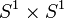$S^1 \times S^1$. The 2-torus is also denoted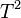$T^2$.

The term torus more generally refers to a product of finitely many copies of the circle, equipped with the product topology. The$n$-torus is sometimes denoted as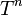$T^n$.

### As a subspace of$\R^3$

A 2-torus in$\R^3$ is obtained as the surface of revolution achieved by revolving a circle about a line in its plane that does not intersect it.

## Topological space properties

Property Satisfied? Is the property a homotopy-invariant property of topological spaces? Explanation Corollary properties satisfied/dissatisfied
manifold Yes No product of manifolds is manifold -- it is a product of two circles. Also, it can be embedded as a closed submanifold in$\R^3$. satisfies: metrizable space, second-countable space, and all the separation axioms down from perfectly normal space and monotonically normal space, including normal, completely regular, regular, Hausdorff, etc.
path-connected space Yes Yes path-connectedness is product-closed -- it is a product of circles, which are path-connected spaces. satisfies: connected space, connected manifold, homogeneous space (via connected manifold, see connected manifold implies homogeneous)
simply connected space No Yes The circle isn't simply connected, and fundamental group of product is product of fundamental groups. dissatisfies: simply connected manifold
rationally acyclic space No Yes The second homology group is isomorphic to the group of integers, hence it is nontrivial and has nontrivial torsion-free part. See homology of torus dissatisfies: acyclic space, weakly contractible space, contractible space
space with Euler characteristic zero Yes Yes Euler characteristic of product is product of Euler characteristics, and the circle has Euler characteristic zero. Alternatively, we can use that Euler characteristic of compact connected nontrivial Lie group is zero. See homology of torus.
space with Euler characteristic one No Yes The Euler characteristic is 0, see above.
compact space Yes No product of circles, which are compact; see Tychonoff's theorem satisfies: compact manifold, compact polyhedron, polyhedron (via compact manifold), compact Hausdorff space, and all properties weaker than compactness

## Algebraic topology

### Homology groups

Further information: homology of torus

The homology groups with coefficients in$\mathbb{Z}$ are as follows: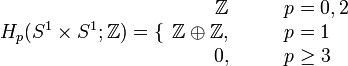$H_p(S^1 \times S^1;\mathbb{Z}) = \lbrace\begin{array}{rl} \mathbb{Z} & \qquad p = 0,2 \\ \mathbb{Z} \oplus \mathbb{Z}, & \qquad p = 1\\0, & \qquad p \ge 3\\\end{array}$

The homology groups with coefficients in a module$M$ are as follows:$H_p(S^1 \times S^1;M) = \lbrace\begin{array}{rl} M & \qquad p = 0,2 \\ M \oplus M, & \qquad p = 1\\0, & \qquad p \ge 3\\\end{array}$

### Cohomology groups

Further information: cohomology of torus

The cohomology group with coefficients in$\mathbb{Z}$ are as follows: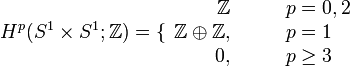$H^p(S^1 \times S^1;\mathbb{Z}) = \lbrace\begin{array}{rl} \mathbb{Z} & \qquad p = 0,2 \\ \mathbb{Z} \oplus \mathbb{Z}, & \qquad p = 1\\0, & \qquad p \ge 3\\\end{array}$

The cohomology groups with coefficients in a module$M$ are as follows: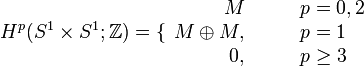$H^p(S^1 \times S^1;\mathbb{Z}) = \lbrace\begin{array}{rl} M & \qquad p = 0,2 \\ M \oplus M, & \qquad p = 1\\0, & \qquad p \ge 3\\\end{array}$

### Homology-based invariants

Invariant General description Description of value for torus$T^n = (S^1)^n$ Description of value for torus$T^2 = S^1 \times S^1$
Betti numbers The$k^{th}$ Betti number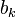$b_k$ is the rank of the$k^{th}$ homology group.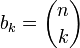$b_k = \binom{n}{k}$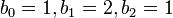$b_0 = 1, b_1 = 2, b_2 = 1$, all higher$b_k$ are zero.
Poincare polynomial Generating polynomial for Betti numbers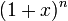$(1 + x)^n$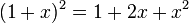$(1 + x)^2 = 1 + 2x + x^2$
Euler characteristic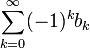$\sum_{k=0}^\infty (-1)^k b_k$, equal to the Poincare polynomial evaluated at -1. 0 (hence it is a space with Euler characteristic zero). This can also be seen from the fact that we have a group (see Euler characteristic of compact connected nontrivial Lie group is zero) or from the fact that it is a product of circles, and the Euler characteristic of the circle is zero (see Euler characteristic of product is product of Euler characteristics). 0

### Homotopy groups

Further information: homotopy of torus

The homotopy groups are given as follows:

Value of$k$ General name for homotopy group or set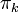$\pi_k$ Value of$\pi_k(S^1 \times S^1)$
0 set of path components one-point set. With the interpretation of the space as a H-space, we can view this as the trivial group
1 fundamental group$\mathbb{Z} \times \mathbb{Z}$, i.e., the free abelian group of rank two. This follows from the fact that fundamental group of product is product of fundamental groups, and the fundamental group of the circle being$\mathbb{Z}$.$k \ge 2$ higher homotopy group trivial group

## Algebraic and coalgebraic structure

### Algebraic structure

The 2-torus can be given the structure of a topological group, arising as the external direct product of two copies of the circle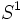$S^1$, which itself is a group (for instance, it has a group structure if identified with the set of complex numbers of modulus 1). With this structure, the 2-torus is an abelian group.

On account of being a topological group, the 2-torus also acquires the structure of a H-space. Some consequences are:

• The zeroth homotopy set naturally acquires a group structure now -- in this case the trivial group.
• The fundamental group must be an abelian group, and hence must be isomorphic to the first homology group. In this case, both groups are isomorphic to$\mathbb{Z}^2$, the free abelian group of rank two.

### Coalgebraic structure

Is there a coalgebraic structure?

## Manifold-type invariants

Invariant Value Explanation
dimension (we can use any of the dimension definitions since this is a connected manifold) 2
smallest dimension of Euclidean space in which it can be immersed 3 The usual embedding in$\R^3$ obtained by taking the surface of revolution of a circle.
smallest dimension of Euclidean space in which it can be embedded 3 The usual embedding in$\R^3$ obtained by taking the surface of revolution of a circle.
smallest dimension of Euclidean space in which it can be embedded as a flat submanifold 4 Consider the usual embedding of$S^1$ in$\R^2$ as a unit circle. This is an embedding as a flat submanifold. Consider$\R^4$ as the product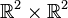$\R^2 \times \R^2$ and consider the induced embedding of$S^1 \times S^1$ in it.
minimum number of charts needed in an atlas for this manifold 3 Pick a point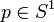$p \in S^1$. Then, the subsets$S^1 \times (S^1 \setminus \{ p \})$,$(S^1 \setminus \{ p \}) \times S^1$, and a small open neighborhood of the point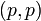$(p,p)$, together form a collection of Euclidean open neighborhoods that cover the manifold.